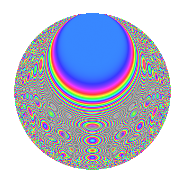# Properties

 Label 403.2.yLevel 403 Weight 2 Character orbit y Rep. character $$\chi_{403}(64,\cdot)$$ Character field $$\Q(\zeta_{10})$$ Dimension 136 Newforms 1 Sturm bound 74 Trace bound 0

# Related objects

## Defining parameters

 Level: $$N$$ = $$403 = 13 \cdot 31$$ Weight: $$k$$ = $$2$$ Character orbit: $$[\chi]$$ = 403.y (of order $$10$$ and degree $$4$$) Character conductor: $$\operatorname{cond}(\chi)$$ = $$403$$ Character field: $$\Q(\zeta_{10})$$ Newforms: $$1$$ Sturm bound: $$74$$ Trace bound: $$0$$

## Dimensions

The following table gives the dimensions of various subspaces of $$M_{2}(403, [\chi])$$.

Total New Old
Modular forms 152 152 0
Cusp forms 136 136 0
Eisenstein series 16 16 0

## Trace form

 $$136q - 10q^{3} + 22q^{4} - 32q^{9} + O(q^{10})$$ $$136q - 10q^{3} + 22q^{4} - 32q^{9} + 6q^{10} + 48q^{12} - 16q^{13} - 4q^{14} - 46q^{16} + 12q^{17} - 44q^{22} + 16q^{23} - 92q^{25} - 48q^{26} - 52q^{27} - 10q^{29} + 28q^{35} - 62q^{38} - 31q^{39} + 12q^{40} + 22q^{42} - 66q^{43} + 64q^{48} + 58q^{49} + 28q^{51} + 27q^{52} + 44q^{55} - 208q^{56} + 4q^{61} + 72q^{62} + 10q^{64} + 7q^{65} + 38q^{66} + 44q^{68} + 46q^{69} + 44q^{74} + 34q^{75} + 48q^{77} + 5q^{78} + 48q^{79} - 12q^{81} + 8q^{82} - 60q^{87} - 160q^{88} + 142q^{90} - 80q^{91} + 28q^{92} - 108q^{94} + 60q^{95} + O(q^{100})$$

## Decomposition of $$S_{2}^{\mathrm{new}}(403, [\chi])$$ into irreducible Hecke orbits

Label Dim. $$A$$ Field CM Traces $q$-expansion
$$a_2$$ $$a_3$$ $$a_5$$ $$a_7$$
403.2.y.a $$136$$ $$3.218$$ None $$0$$ $$-10$$ $$0$$ $$0$$